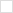This wiki is locked. Future workgroup activity and specification development must take place at our new wiki. For more information, see this blog post about the new governance model and this post about changes to the website.
TWiki>Main Web>PmHome>MetricsHome>MetricsWorkingDocuments>MetricsEMS10RestApi>MetricsEMS10RestApiDataModel>MetricsEmsTriangularDistribution (24 Sep 2010, ArthurRyman)

# ems:TriangularDistribution

## Description

Consider how software developers typically express estimates for size or effort. For example, when asked how long a programming task will take, a developer will often say something like, "It usually takes me 2 days to complete that type of work. If everything goes perfectly, I might be able to do it in 1 day. However, if I hit problems, it might take as long as 5 days." The structure of this estimate consists of 3 values, e.g. the realistic estimate of 2 days, the optimistic estimate of 1 day, and the pessimistic estimate of 5 days.

We can give this estimate a probabilistic interpretation as follows. Let's assume that the value, e.g. size, duration, or effort, we are estimating is a real number that can vary continuously over some range. The probability is zero below some minumum value, rises linearly to some peak value, then drops linearly to zero again at some maximum value. The shape of this distribution is a triangular and we call this a triangular distribution. Rather than use terms like optimistic and pessimistic which imply that some values are more desirable than others, we use the neutral terms: low, most likely, and high to describe the three values that define the triangular distribution.

An ems:TriangularDistribution resource represents a triangular distribution.

## Properties

An ems:TriangularDistribution resource has the following properties:

Property Range Type Occurrence Edits Description
ems:low datatype xsd:double exactly-one read-write The low value.
ems:mostLikely datatype xsd:double exactly-one read-write The most likely value.
ems:high datatype xsd:double exactly-one read-write The high value.

### ems:low

This is the low value of the distribution. The probability is zero below this value and begins to go positive above this value.

### ems:mostLikely

This is the most likely value of the distribution. The probability is a maximum at this value. This value MUST be greater than the value of ems:low.

### ems:high

This is the high value of the distribution. The probability is zero above this value and begins to go positive below this value.

## Examples

### Triangular Distribution 1

The following resource represents a triangular distribution with a low value of 30000, a most likely value of 50000, and a high value of 100000:

###### Listing of Triangular Distribution 1
```<?xml version="1.0"?>
<ems:TriangularDistribution xmlns:ems="http://open-services.net/ns/ems#"
xmlns:rdf="http://www.w3.org/1999/02/22-rdf-syntax-ns#">
<ems:low rdf:datatype="http://www.w3.org/2001/XMLSchema#double">30000</ems:low>
<ems:mostLikely rdf:datatype="http://www.w3.org/2001/XMLSchema#double">50000</ems:mostLikely>
<ems:high rdf:datatype="http://www.w3.org/2001/XMLSchema#double">100000</ems:high>
</ems:TriangularDistribution>
```Български Cesky Dansk Deutsch English Español Français Italiano 日本語 Nederlands Polski Português Русский Svenska 简体中文 簡體中文